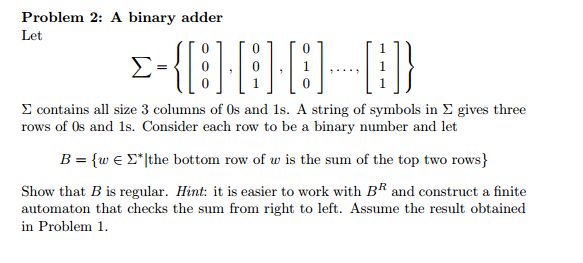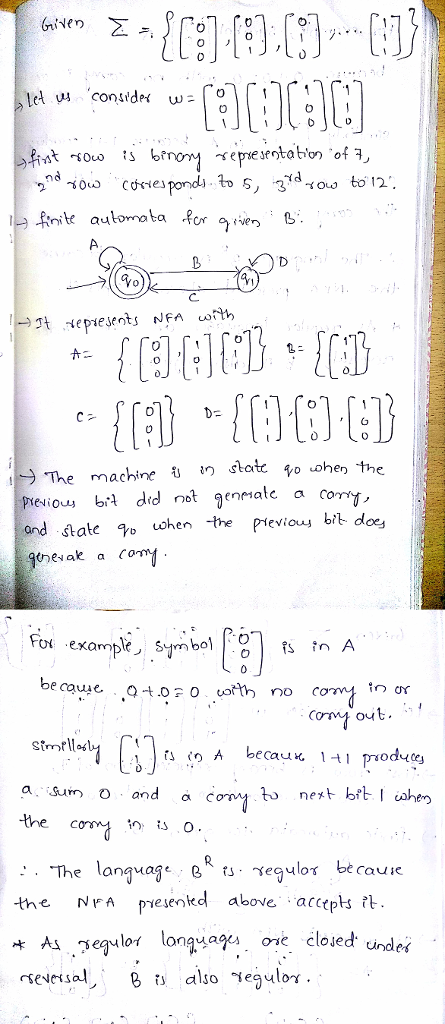# Question & Answer: Problem 2: A binary adder Let 0 Σ contains all size 3 columns of 0s and 1s. A string of symbols in Σ gives three rows of Os and 1s…..Problem 2: A binary adder Let 0 Σ contains all size 3 columns of 0s and 1s. A string of symbols in Σ gives three rows of Os and 1s. Consider each row to be a binary number and let B = {w ε Σ.[the bottom row of w is the sum of the top two rows) Show that B is regular. Hint: it is easier to work with B and construct a finite automaton that checks the sum from right to left. Assume the result obtained in Problem 1